# 四、特征缩放的效果：从词袋到 TF-IDF

## Tf-Idf: 词袋的小转折

Tf-Idf 是词袋的一个小小的转折。它表示词频-逆文档频。tf-idf不是查看每个文档中每个单词的原始计数，而是查看每个单词计数除以出现该单词的文档数量的标准化计数。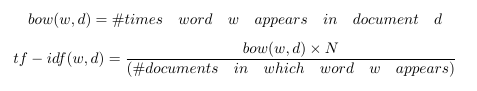N代表数据集中所有文档的数量。分数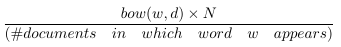就是所谓的逆文件频率。如果一个单词出现在许多文档中，则其逆文档频率接近1。如果单词出现在较少文档中，则逆文档频率要高得多。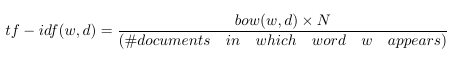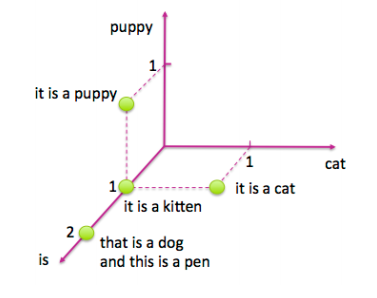## Tf-Idf的含义

Tf-idf使罕见的单词更加突出，并有效地忽略了常见单词。

## 测试

Tf-idf通过乘以一个常量来转换字数统计特性。因此，它是特征缩放的一个例子，这是第2章介绍的一个概念。特征缩放在实践中效果有多好？ 我们来比较简单文本分类任务中缩放和未缩放特征的表现。 coding时间到！

### 样例4-1：使用python加载和清洗Yelp评论数据集

import json
import pandas as pd
biz_f =
ess.json')
biz_f.close()
review_file.close()
# Pull out only Nightlife and Restaurants businesses
two_biz = biz_df[biz_df.apply(lambda x: 'Nightlife' in x['categories']
or 'Restaurants' in x['categories'],axis=1)]
# Join with the reviews to get all reviews on the two types of business
# Trim away the features we won't use
'name',
'stars_y',
'text',
'categories']]
# Create the target column--True for Nightlife businesses, and False otherwise
two_biz_reviews['target'] = twobiz_reviews.apply(lambda x: 'Nightlife' in
x['categories'],axis=1)


## 建立分类数据集

1. 随机抽取10%夜生活场所评论以及2.1%的餐厅评论（选取合适的百分比使得每个种类的数目大致一样）

2. 将数据集分成比例为7：3的训练集和测试集。在这个例子里，训练集包括29，264条评论，测试集有12542条。

3. 训练数据包括46，924个不同的单词，这是词袋表示中特征的数量。

### 样例4-2：创建一个分类数据集

# Create a class-balanced subsample to play with
nightlife = twobiz_reviews[twobiz_reviews.apply(lambda x: 'Nightlife' in
x['categories'], axis=1)]
restaurants = twobiz_reviews[twobiz_reviews.apply(lambda x: 'Restaurants' in
x['categories'], axis=1)]
nightlife_subset = nightlife.sample(frac=0.1, random_state=123)
restaurant_subset = restaurants.sample(frac=0.021, random_state=123)
combined = pd.concat([nightlife_subset, restaurant_subset])
# Split into training and test data sets
training_data, test_data = modsel.train_test_split(combined,
train_size=0.7,
random_state=123)
training_data.shape
# (29264, 5)
test_data.shape
# (12542, 5)



## 测试集上进行特征缩放

### 样例4-3：特征变换

# Represent the review text as a bag-of-words
bow_transform = text.CountVectorizer()
X_tr_bow = bow_transform.fit_transform(training_data['text'])
X_te_bow = bow_transform.transform(test_data['text'])
len(bow_transform.vocabulary_)
# 46924
y_tr = training_data['target']
y_te = test_data['target']
# Create the tf-idf representation using the bag-of-words matrix
tfidf_trfm = text.TfidfTransformer(norm=None)
X_tr_tfidf = tfidf_trfm.fit_transform(X_tr_bow)
X_te_tfidf = tfidf_trfm.transform(X_te_bow)
# Just for kicks, l2-normalize the bag-of-words representation
X_tr_l2 = preproc.normalize(X_tr_bow, axis=0)
X_te_l2 = preproc.normalize(X_te_bow, axis=0)



## 使用逻辑回归进行分类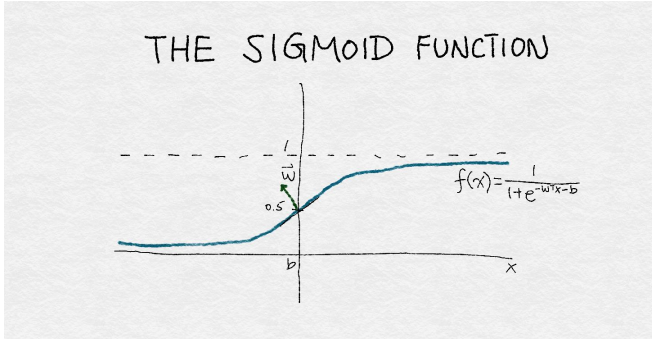### 样例4-4：使用默认参数训练逻辑回归分类器

def simple_logistic_classify(X_tr, y_tr, X_test, y_test, description):
## Helper function to train a logistic classifier and score on test data
m = LogisticRegression().fit(X_tr, y_tr)
s = m.score(X_test, y_test)
print ('Test score with', description, 'features:', s)
return m
m1 = simple_logistic_classify(X_tr_bow, y_tr, X_te_bow, y_te, 'bow')
m2 = simple_logistic_classify(X_tr_l2, y_tr, X_te_l2, y_te, 'l2-normalized')
m3 = simple_logistic_classify(X_tr_tfidf, y_tr, X_te_tfidf, y_te, 'tf-idf')
# Test score with bow features: 0.775873066497
# Test score with l2-normalized features: 0.763514590974
# Test score with tf-idf features: 0.743182905438



## 重点：比较模型时调整超参数

##### Table4-1.对夜场和餐厅的Yelp评论进行逻辑回归的最佳参数设置

Method L2 Regularization
BOW 0.1
L2-normalized 10
TF-IDF 0.01

### 样例4-5：使用网格搜索调整逻辑回归超参数

>>> import sklearn.model_selection as modsel# Specify a search grid, then do 5-fold grid search for each of the feature sets
>>> param_grid_ = {'C': [1e-5, 1e-3, 1e-1, 1e0, 1e1, 1e2]# Tune classifier for bag-of-words representation
>>> bow_search = modsel.GridSearchCV(LogisticRegression(), cv=5,
param_grid=param_grid_)
>>> bow_search.fit(X_tr_bow, y_tr)# Tune classifier for L2-normalized word vector
>>> l2_search = modsel.GridSearchCV(LogisticRegression(), cv=5,
param_grid=param_grid_)
>>> l2_search.fit(X_tr_l2, y_tr)# Tune classifier for tf-idf
>>> tfidf_search = modsel.GridSearchCV(LogisticRegression(), cv=5,
param_grid=param_grid_)
>>> tfidf_search.fit(X_tr_tfidf, y_tr)
# Let's check out one of the grid search outputs to see how it went
>>> bow_search.cv_results_{'mean_fit_time':
array([ 0.43648252, 0.94630651, 5.64090128, 15.31248307,
31.47010217, 42.44257565]),
'mean_score_time':
array([ 0.00080056, 0.00392466, 0.00864897, 0.00784755, 0.01192751,
0.0072515 ]),
'mean_test_score':
array([ 0.57897075, 0.7518111 , 0.78283898, 0.77381766, 0.75515992,
0.73937261]),
'mean_train_score':
array([ 0.5792185 , 0.76731652, 0.87697341, 0.94629064, 0.98357195,
0.99441294]),
'param_C': masked_array(data = [1e-05 0.001 0.1 1.0 10.0 100.0],
mask = [False False False False False False],
fill_value = ?),
'params': ({'C': 1e-05},
{'C': 0.001},
{'C': 0.1},
{'C': 1.0},
{'C': 10.0},
{'C': 100.0}),
'rank_test_score': array([6, 4, 1, 2, 3, 5]),
'split0_test_score':
array([ 0.58028698, 0.75025624, 0.7799795 , 0.7726341 , 0.75247694,
0.74086095]),
'split0_train_score':
array([ 0.57923964, 0.76860316, 0.87560871, 0.94434003, 0.9819308 ,
0.99470312]),
'split1_test_score':
array([ 0.5786776 , 0.74628396, 0.77669571, 0.76627371, 0.74867589,
0.73176149]),
'split1_train_score':
array([ 0.57917218, 0.7684849 , 0.87945837, 0.94822946, 0.98504976,
0.99538678]),
'split2_test_score':
array([ 0.57816504, 0.75533914, 0.78472578, 0.76832394, 0.74799248,
0.7356911 ]),
'split2_train_score':
array([ 0.57977019, 0.76613558, 0.87689548, 0.94566657, 0.98368288,
0.99397719]),
'split3_test_score':
array([ 0.57894737, 0.75051265, 0.78332194, 0.77682843, 0.75768968,
0.73855092]),
'split3_train_score':
array([ 0.57914745, 0.76678626, 0.87634546, 0.94558346, 0.98385443,
0.99474628]),
'split4_test_score':
array([ 0.57877649, 0.75666439, 0.78947368, 0.78503076, 0.76896787,
0.75 ]),
'split4_train_score':
array([ 0.57876303, 0.7665727 , 0.87655903, 0.94763369, 0.98334188,
0.99325132]),
'std_fit_time':
array([ 0.03874582, 0.02297261, 1.18862097, 1.83901079, 4.21516797,
2.93444269]),
'std_score_time':
array([ 0.00160112, 0.00605009, 0.00623053, 0.00698687, 0.00713112,
0.00570195]),
'std_test_score':
array([ 0.00070799, 0.00375907, 0.00432957, 0.00668246, 0.00771557,
0.00612049]),
'std_train_score':
array([ 0.00032232, 0.00102466, 0.00131222, 0.00143229, 0.00100223,
0.00073252])}
########
# Plot the cross validation results in a box-and-whiskers plot to
# visualize and compare classifier performance
########
>>> search_results = pd.DataFrame.from_dict({'bow':
bow_search.cv_results_['mean_test_score'],
'tfidf':
tfidf_search.cv_results_['mean_test_score'],
'l2':
l2_search.cv_results_['mean_test_score']})
# Our usual matplotlib incantations. Seaborn is used here to make
# the plot pretty
>>> import matplotlib.pyplot as plt
>>> import seaborn as sns
>>> sns.set_style("whitegrid")
>>> ax = sns.boxplot(data=search_results, width=0.4)
>>> ax.set_ylabel('Accuracy', size=14)
>>> ax.tick_params(labelsize=14)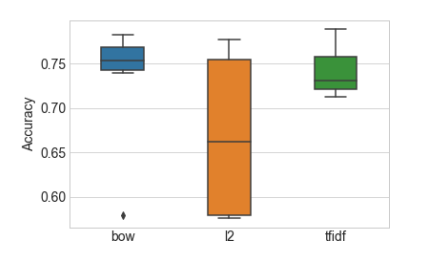##### Table4-2.每个超参数设置的平均交叉验证分类器准确度。 星号表示最高精度

Regularization Parameter BOW normalized Tf-idf
0.00001 0.578971 0.575724 0.721638
0.001 0.751811 0.575724 0.788648*
0.1 0.782839* 0.589120 0.763566
1 0.773818 0.734247 0.741150
10 0.755160 0.776756* 0.721467
100 0.739373 0.761106 0.712309

### 示例4-6：最终的训练和测试步骤来比较不同的特征集

# Train a final model on the entire training set, using the best hyperparamete
# settings found previously. Measure accuracy on the test set.
>>> m1 = simple_logistic_classify(X_tr_bow, y_tr, X_te_bow, y_te, 'bow', _C=bow_search.best_params_['C'])
>>> m2 = simple_logistic_classify(X_tr_l2, y_tr, X_te_l2, y_te, 'l2-normalized', _C=l2_search.best_params_['C'])
>>> m3 = simple_logistic_classify(X_tr_tfidf, y_tr, X_te_tfidf, y_te, 'tf-idf', _C=tfidf_search.best_params_['C'])
Test score with bow features: 0.78360708021
Test score with l2-normalized features: 0.780178599904
Test score with tf-idf features: 0.788470738319


##### Table4-3.BOW, Tf-Idf,以及L2正则化的最终分类精度

Feature Set Test Accuracy
Bag-of-Words 0.78360708021
L2 -normalized 0.780178599904
Tf-Idf 0.788470738319

## 深入：发生了什么？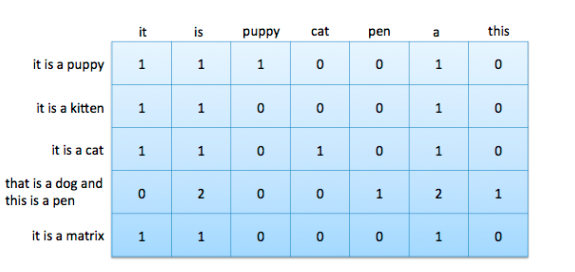## Tf-idf=列缩放

Tf-idf和L2归一化都是数据矩阵上的列操作。 正如附录A所讨论的那样，训练线性分类器归结为寻找最佳的线性组合特征，这是数据矩阵的列向量。 解空间的特征是列空间和数据矩阵的空间。训练过的线性分类器的质量直接取决于数据矩阵的零空间和列空间。 大的列空间意味着特征之间几乎没有线性相关性，这通常是好的。 零空间包含“新”数据点，不能将其表示为现有数据的线性组合; 大的零空间可能会有问题。（强烈建议希望对诸如线性决策表面，特征分解和矩阵的基本子空间等概念进行的回顾的读者阅读附录A。)

## 总结

Strang, Gilbert. 2006. Linear Algebra and Its Applications. Brooks Cole Cengage, fourth edition.

$$^1$$ 严格地说，矩阵矩阵的行空间和列空间不能都是满秩的。 两个子空间的最大秩是m（行数）和n（列数）中的较小者。 这就是我们所说的满秩。